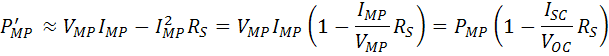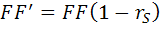# Series Resistance

Series resistance in a solar cell has three causes: firstly, the movement of current through the emitter and base of the solar cell; secondly, the contact resistance between the metal contact and the silicon; and finally the resistance of the top and rear metal contacts. The main impact of series resistance is to reduce the fill factor, although excessively high values may also reduce the short-circuit current.Schematic of a solar cell with series resistance.

$I={I}_{L}-{I}_{0}\mathrm{exp}\left[\frac{q\left(V+I{R}_{S}\right)}{nkT}\right]$

where: I is the cell output current, IL is the light generated current, V is the voltage across the cell terminals, T is the temperature, q and k are constants, n is the ideality factor, and RS is the cell series resistance. The formula is an example of an implicit function due to the appearance of the current, I, on both sides of the equation and requires numerical methods to solve.

The effect of the series resistance on the IV curve is shown below. To generate the plot the voltage across the diode is varied thereby avoiding the need to solve an implicit equation.

X
020

The effect of series resistance on fill factor. The area of the solar cell is 1 cm2 so that the units of resistance can be either ohm or ohm cm2. The short circuit current (ISC) is unaffected b the series resistance until it is very large.

Series resistance does not affect the solar cell at open-circuit voltage since the overall current flow through the solar cell, and therefore through the series resistance is zero. However, near the open-circuit voltage, the IV curve is strongly affected by the series resistance. A straight-forward method of estimating the series resistance from a solar cell is to find the slope of the IV curve at the open-circuit voltage point.

An equation for the FF as a function of series resistance can be determined by noting that for moderate values of series resistance, the maximum power may be approximated as the power in the absence of series resistance minus the power lost in the series resistance. The equation for the maximum power from a solar cell then becomes:defining a normalized series resistance as;gives the following equation which approximates the effect of series resistance on the output power of a solar cell;Assuming that the open-circuit voltage and short-circuit current are not affected by the series resistance allows the impact of series resistance on FF to be determined;In the above equation the fill factor which is not affected by series resistance is denoted by FF0 and FF' is called FFS. The equation then becomes;An empirical equation, which is slightly more accurate for the relationship between FF0 and FFS is;which is valid for rs < 0.4 and voc > 10.

The following calculator determines the effect of Rs on the solar cell fill factor. Typical values for area-normalized series resistance are between 0.5 Ωcm2 for laboratory type solar cells and up to 1.3 Ωcm2 for commercial solar cells. The current levels in the solar cell have a major impact on the losses due to series resistance and in the following calculator, examine the impact raising the current has on the FF.

Series Resistance Calculator

###### Results

Cell characteristic resistance, RCH X (ohms)
Normalized Voc, voc X (units)
Normalized series resistance, rs X (units)
Approximate fill factor, FF, with Rs FFapprox X
More accurate FF valid for rs < 0.4 and voc > 10 FFs X